Скачать презентацию 1 10 Magnetically coupled networks EMLAB Transformer

1e3042f63ff0a79be70b68055d8bd996.ppt

• Количество слайдов: 29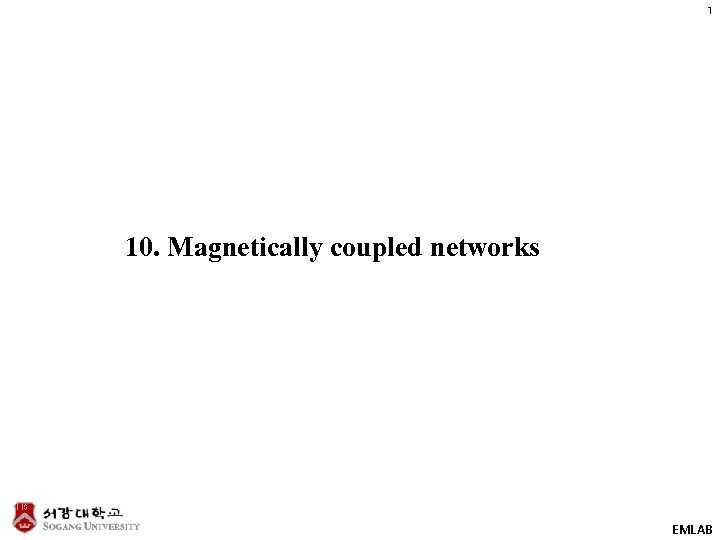1 10. Magnetically coupled networks EMLAB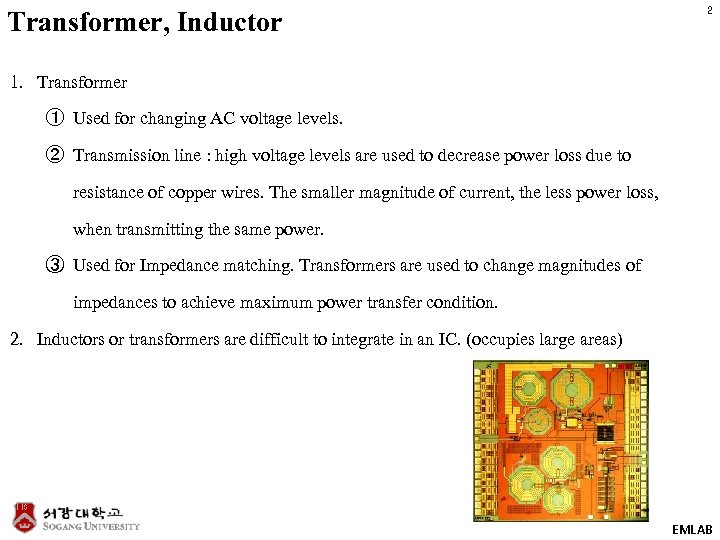Transformer, Inductor 2 1. Transformer ① Used for changing AC voltage levels. ② Transmission line : high voltage levels are used to decrease power loss due to resistance of copper wires. The smaller magnitude of current, the less power loss, when transmitting the same power. ③ Used for Impedance matching. Transformers are used to change magnitudes of impedances to achieve maximum power transfer condition. 2. Inductors or transformers are difficult to integrate in an IC. (occupies large areas) EMLAB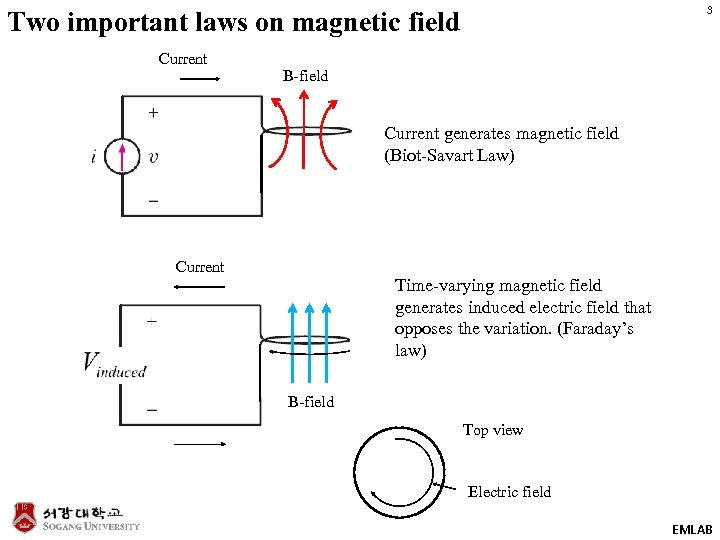3 Two important laws on magnetic field Current B-field Current generates magnetic field (Biot-Savart Law) Current Time-varying magnetic field generates induced electric field that opposes the variation. (Faraday’s law) B-field Top view Electric field EMLAB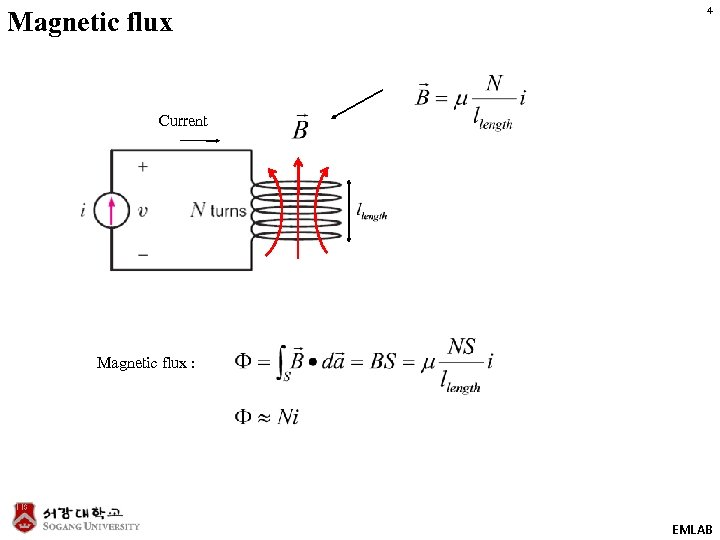Magnetic flux 4 Current Magnetic flux : EMLAB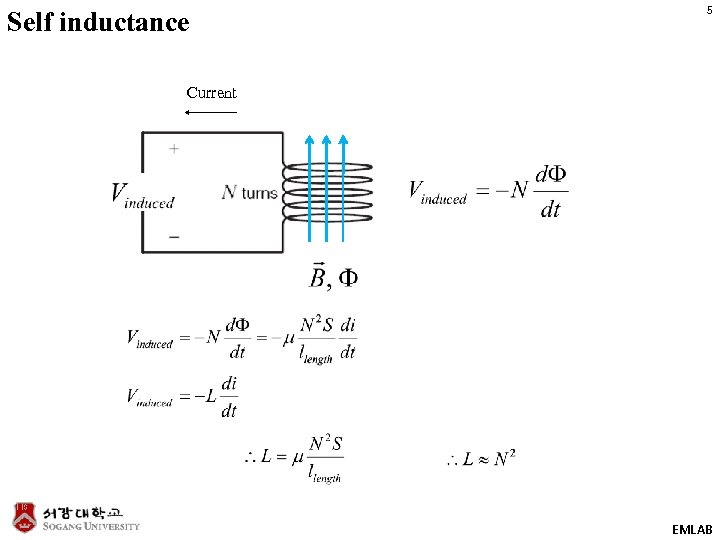Self inductance 5 Current EMLAB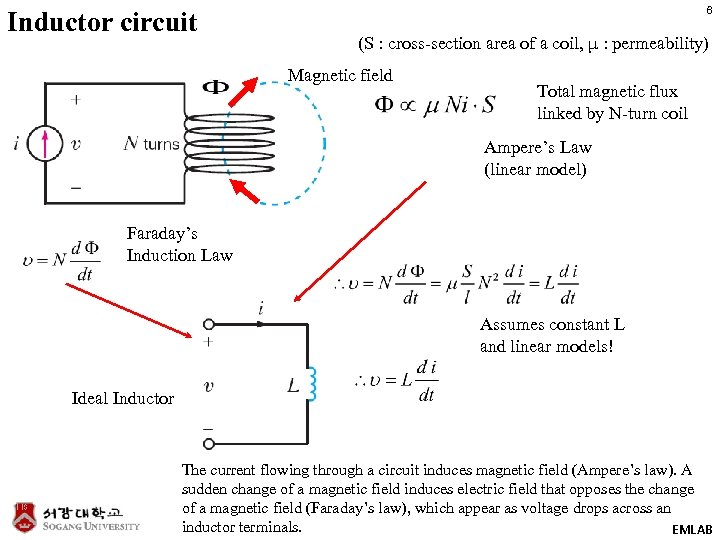Inductor circuit 6 (S : cross-section area of a coil, μ : permeability) Magnetic field Total magnetic flux linked by N-turn coil Ampere’s Law (linear model) Faraday’s Induction Law Assumes constant L and linear models! Ideal Inductor The current flowing through a circuit induces magnetic field (Ampere’s law). A sudden change of a magnetic field induces electric field that opposes the change of a magnetic field (Faraday’s law), which appear as voltage drops across an inductor terminals. EMLAB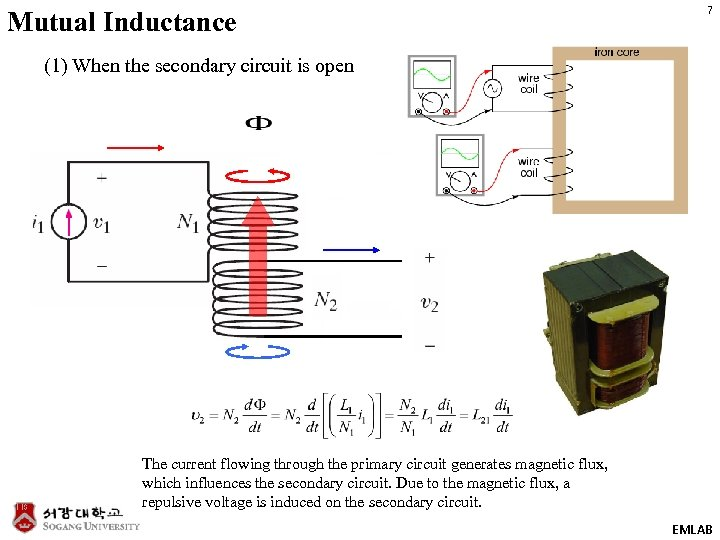Mutual Inductance 7 (1) When the secondary circuit is open The current flowing through the primary circuit generates magnetic flux, which influences the secondary circuit. Due to the magnetic flux, a repulsive voltage is induced on the secondary circuit. EMLAB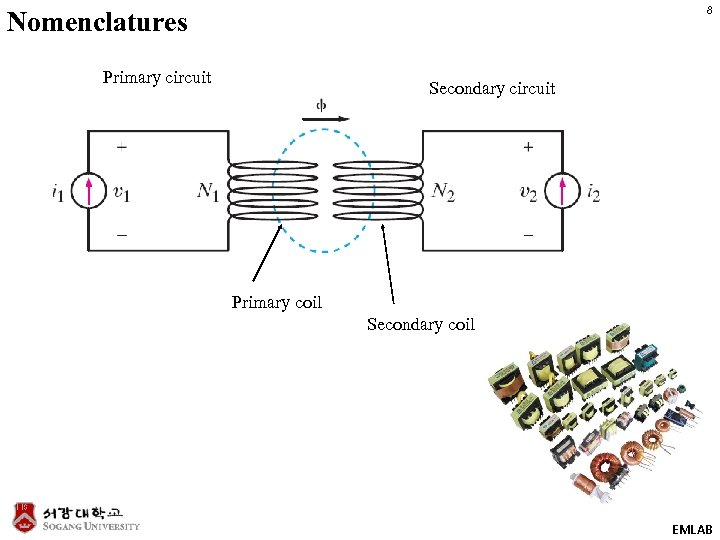8 Nomenclatures Primary circuit Secondary circuit Primary coil Secondary coil EMLAB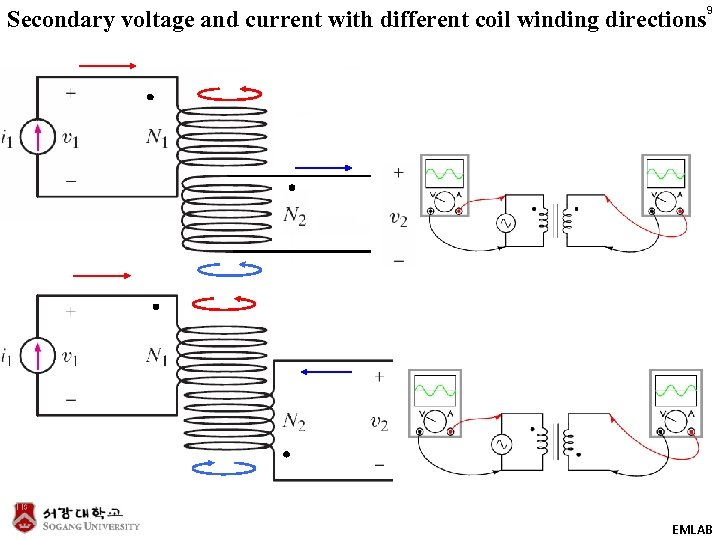Secondary voltage and current with different coil winding directions 9 EMLAB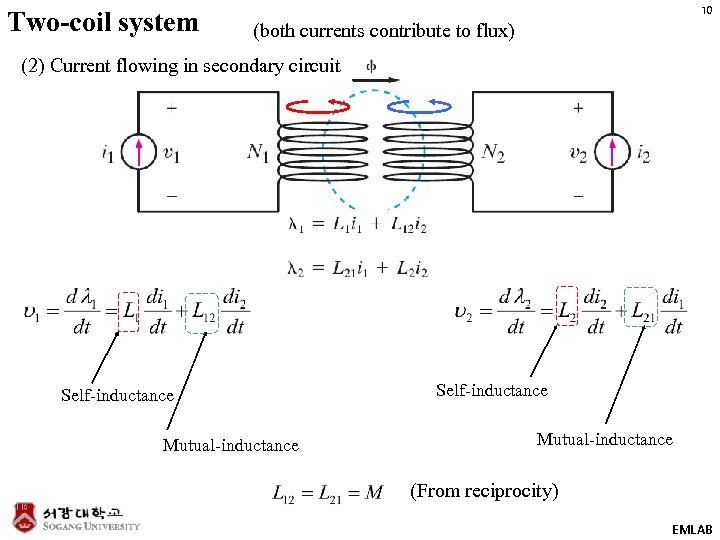Two-coil system 10 (both currents contribute to flux) (2) Current flowing in secondary circuit Self-inductance Mutual-inductance (From reciprocity) EMLAB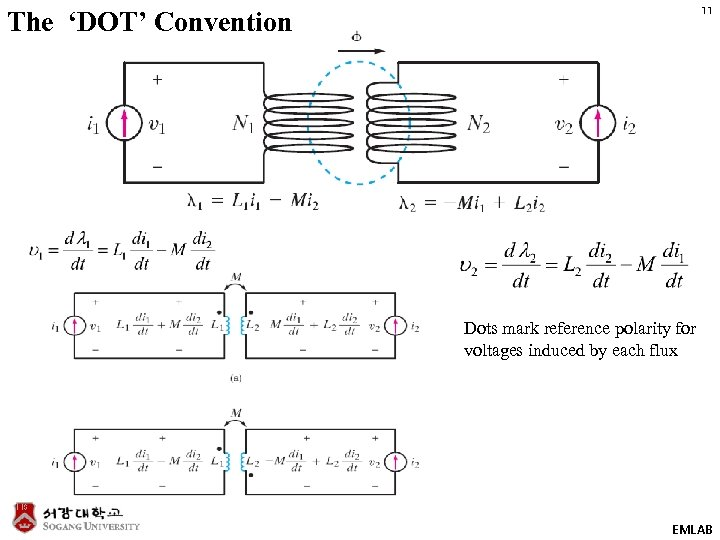11 The ‘DOT’ Convention Dots mark reference polarity for voltages induced by each flux EMLAB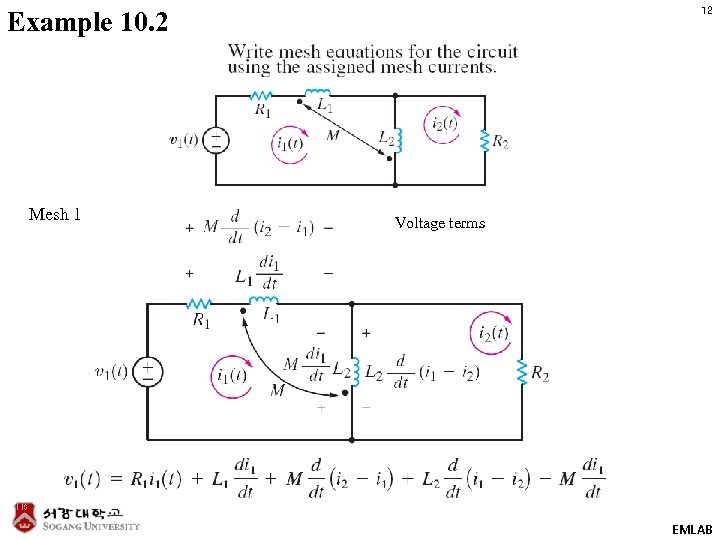12 Example 10. 2 Mesh 1 Voltage terms EMLAB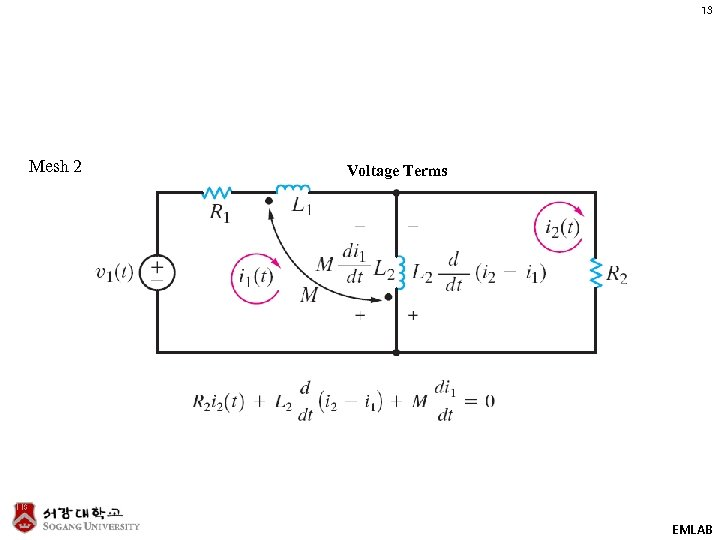13 Mesh 2 Voltage Terms EMLAB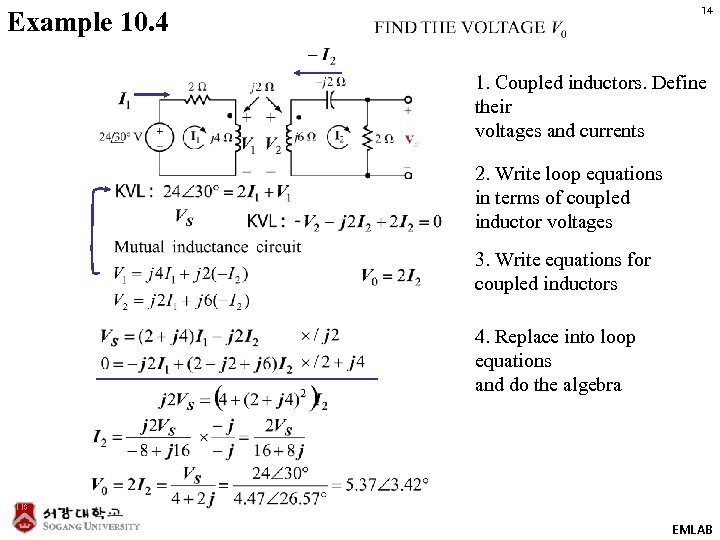14 Example 10. 4 1. Coupled inductors. Define their voltages and currents 2. Write loop equations in terms of coupled inductor voltages 3. Write equations for coupled inductors 4. Replace into loop equations and do the algebra EMLAB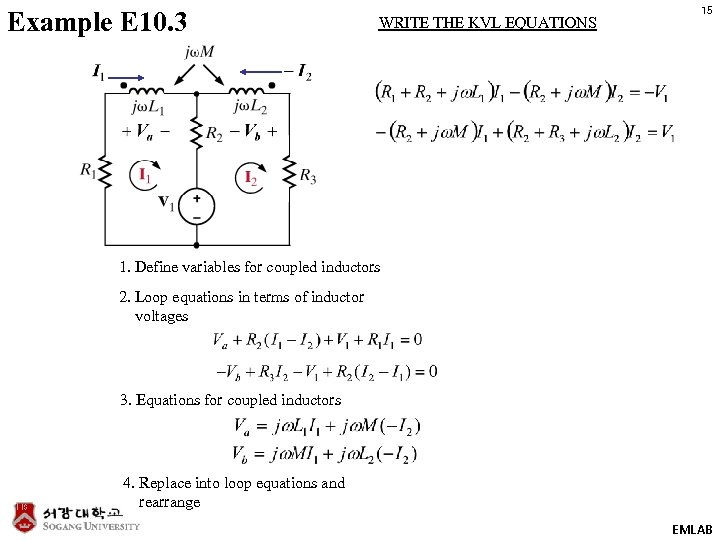Example E 10. 3 WRITE THE KVL EQUATIONS 15 1. Define variables for coupled inductors 2. Loop equations in terms of inductor voltages 3. Equations for coupled inductors 4. Replace into loop equations and rearrange EMLAB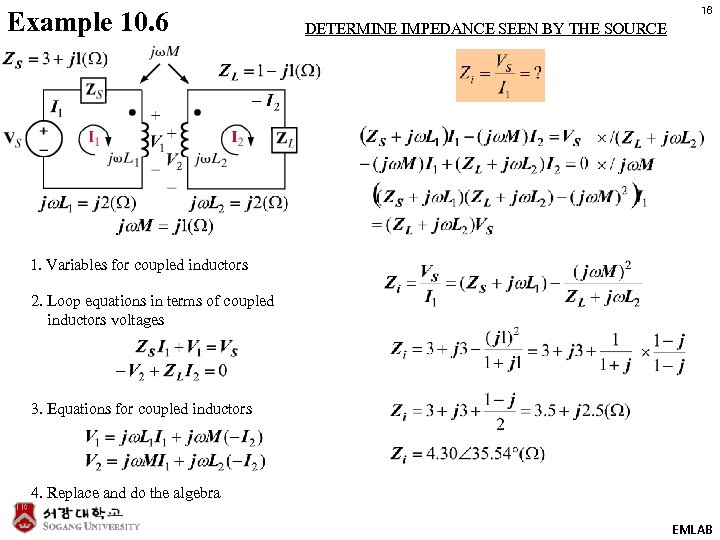Example 10. 6 16 DETERMINE IMPEDANCE SEEN BY THE SOURCE 1. Variables for coupled inductors 2. Loop equations in terms of coupled inductors voltages 3. Equations for coupled inductors 4. Replace and do the algebra EMLAB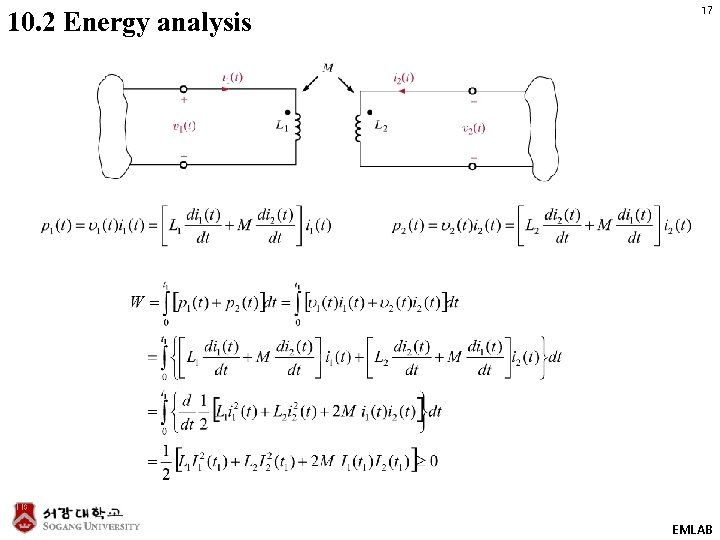10. 2 Energy analysis 17 EMLAB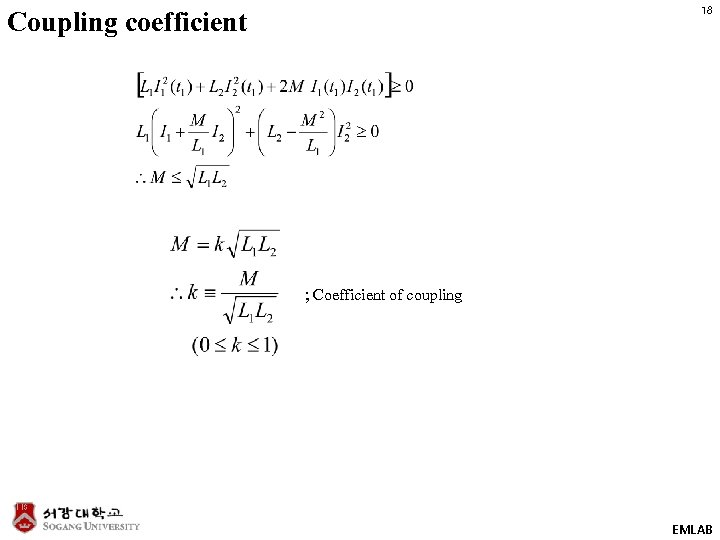18 Coupling coefficient ; Coefficient of coupling EMLAB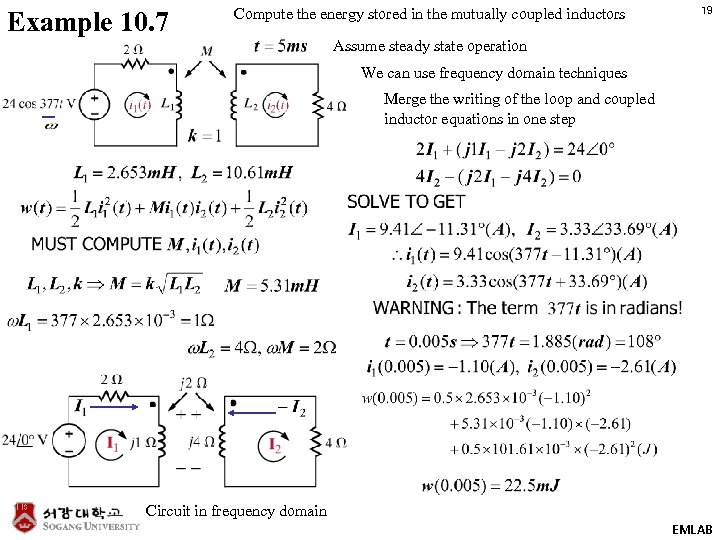Example 10. 7 Compute the energy stored in the mutually coupled inductors 19 Assume steady state operation We can use frequency domain techniques Merge the writing of the loop and coupled inductor equations in one step Circuit in frequency domain EMLAB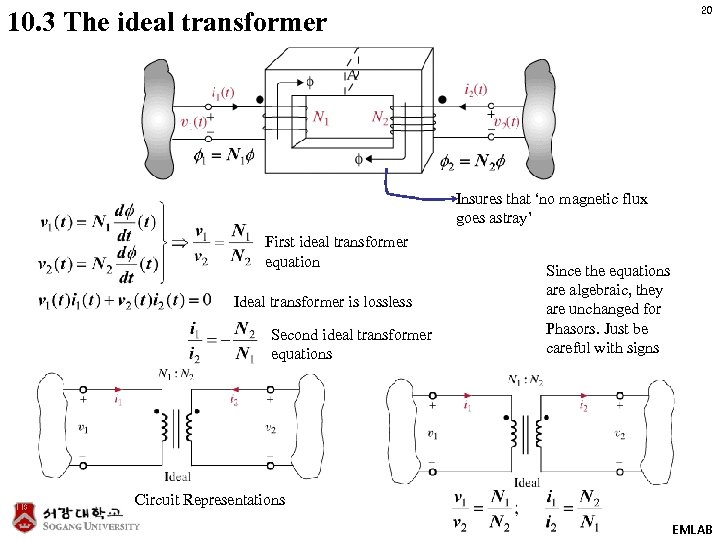20 10. 3 The ideal transformer Insures that ‘no magnetic flux goes astray’ First ideal transformer equation Ideal transformer is lossless Second ideal transformer equations Since the equations are algebraic, they are unchanged for Phasors. Just be careful with signs Circuit Representations EMLAB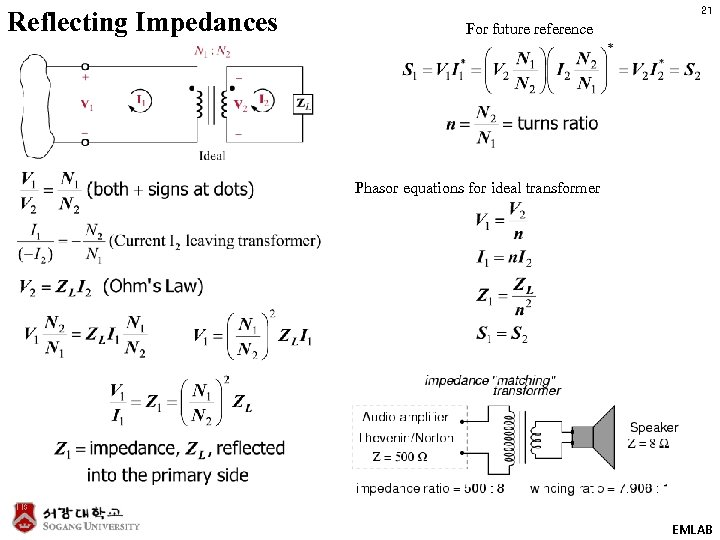Reflecting Impedances 21 For future reference Phasor equations for ideal transformer EMLAB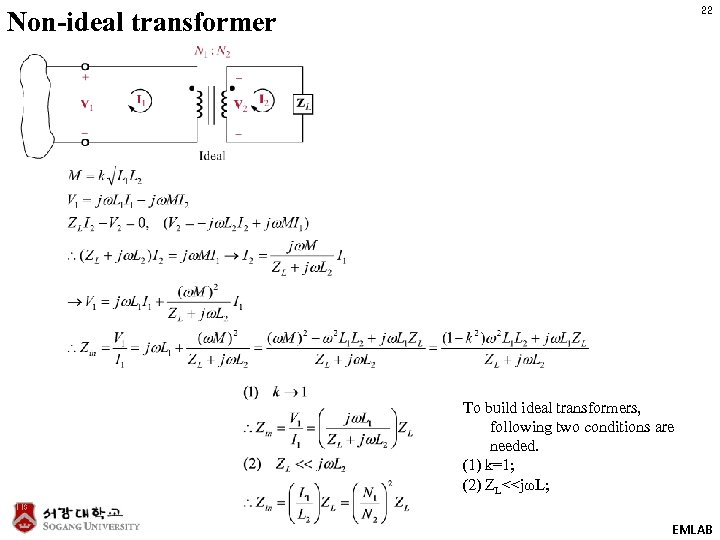22 Non-ideal transformer To build ideal transformers, following two conditions are needed. (1) k=1; (2) ZL<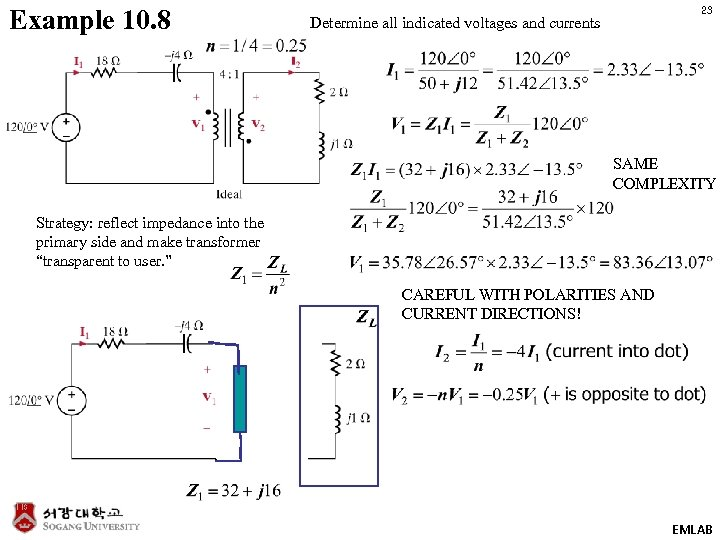Example 10. 8 23 Determine all indicated voltages and currents SAME COMPLEXITY Strategy: reflect impedance into the primary side and make transformer “transparent to user. ” CAREFUL WITH POLARITIES AND CURRENT DIRECTIONS! EMLAB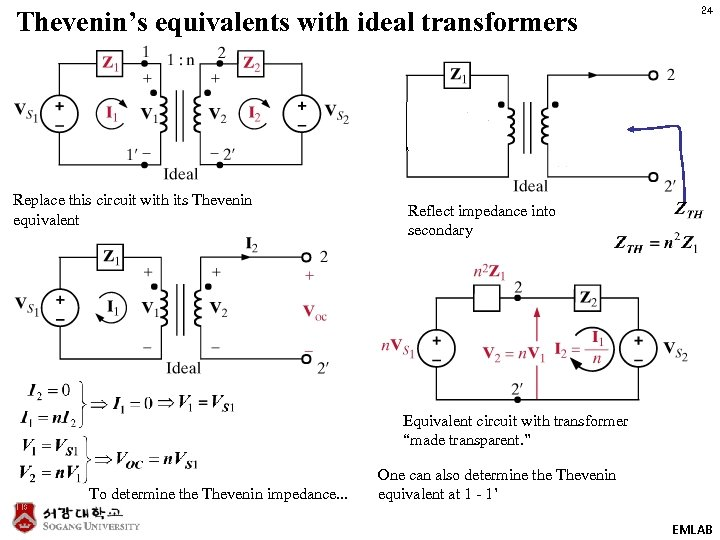Thevenin’s equivalents with ideal transformers Replace this circuit with its Thevenin equivalent 24 Reflect impedance into secondary Equivalent circuit with transformer “made transparent. ” To determine the Thevenin impedance. . . One can also determine the Thevenin equivalent at 1 - 1’ EMLAB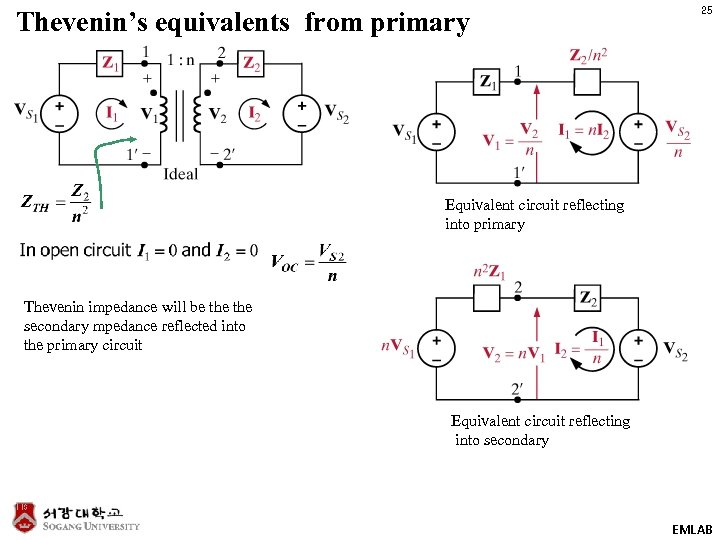Thevenin’s equivalents from primary 25 Equivalent circuit reflecting into primary Thevenin impedance will be the secondary mpedance reflected into the primary circuit Equivalent circuit reflecting into secondary EMLAB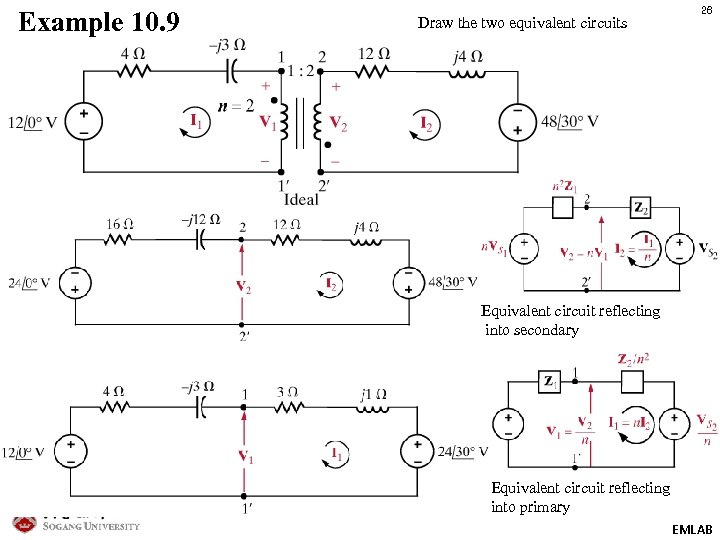Example 10. 9 Draw the two equivalent circuits 26 Equivalent circuit reflecting into secondary Equivalent circuit reflecting into primary EMLAB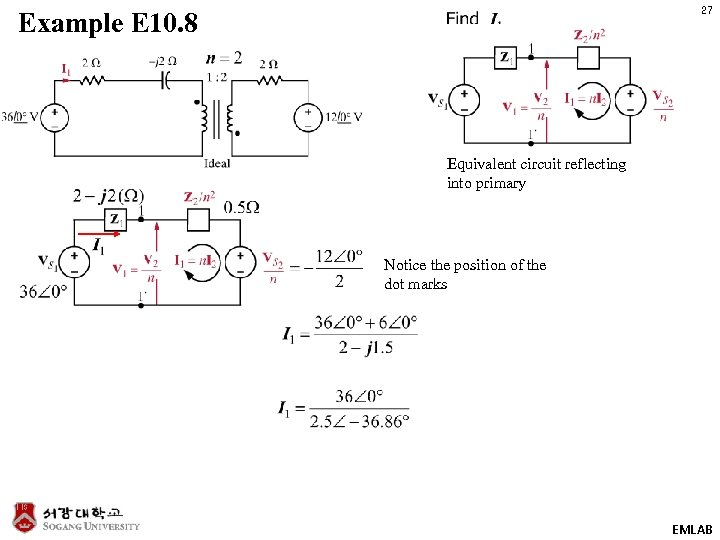27 Example E 10. 8 Equivalent circuit reflecting into primary Notice the position of the dot marks EMLAB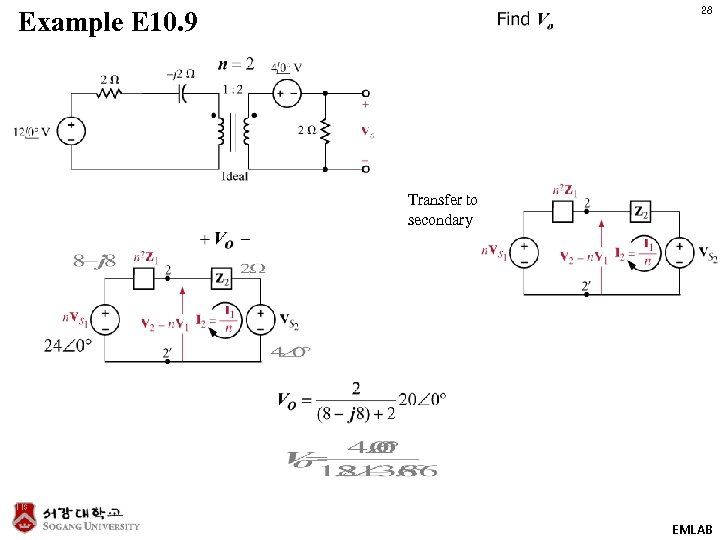28 Example E 10. 9 Transfer to secondary EMLAB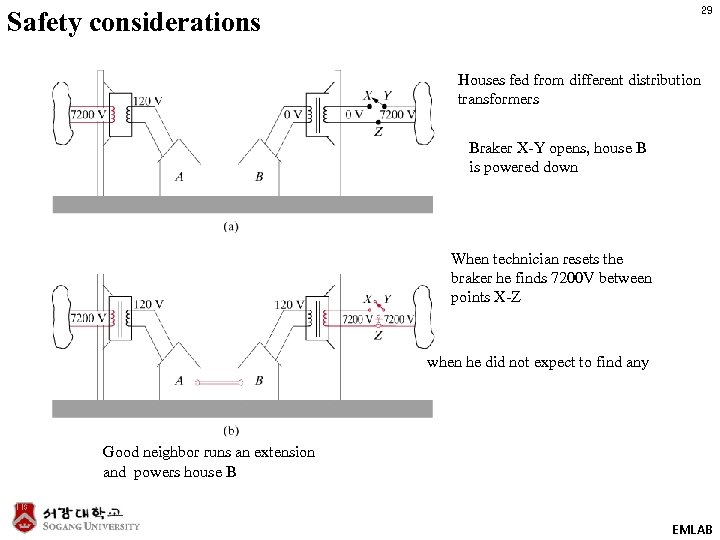29 Safety considerations Houses fed from different distribution transformers Braker X-Y opens, house B is powered down When technician resets the braker he finds 7200 V between points X-Z when he did not expect to find any Good neighbor runs an extension and powers house B EMLAB